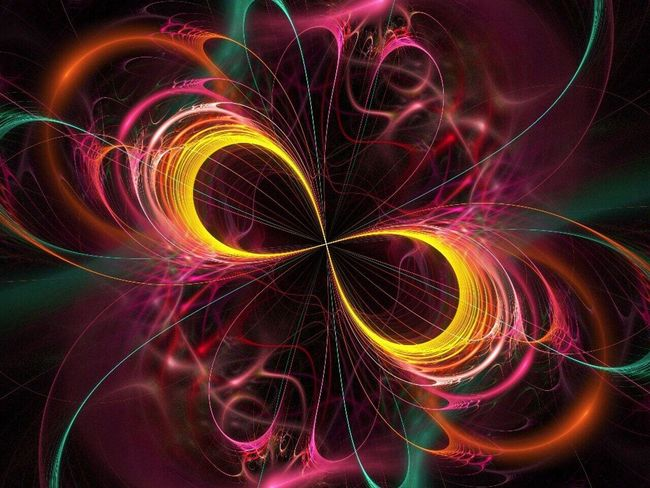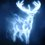# The speaking tree - From Mahashoonya, Opposites AriseToday I realized that little things are infinitely the most important. Read this:

If you have a zero on the left side of an equation, you must have positive and negative quantities on the right side, which cancel each other out. The simplest case is 0 = +5 -5. More complex examples are 0 = +4 +6 -10 and 0 = +7 +4 -5 -6. There can be infinite such equations. In other words, we can say that zero is not really nothing', but it can potentially give rise to infinite possibilities. There is an equation which is not really an equation, 0 = 0, but more about it at the end of this article! Indian spirituality talks aboutmahashoonya', a big zero that precedes and succeeds physical creation. It is nothing' only relative to what we understand bything' in the physical world as perceived by our physical senses. So Brahmn or mahashoonya is nothing like what we know or can perceive. That is why it is shoonya ¬ zero. But yet, it has infinite potentialities which manifest as this magnificent and tremendously varied creation. Just as in the mathematical concept of equation with zero on the left side as mentioned above, this mahashoonya also must create opposites that balance out each other.In the simplest case, if a thing' is created, there has to be ananti-thing' that is equal to and opposite of thing'. In a more complex case, there may be athing' and many antithings' that together are equal to and opposite of thething'.Even more complex case is where there are many things' and manyanti-things' that together cancel each other. In other words, mahashoonya creates a perceiver and the opposite of it ¬ the perceived. The perceiver and the perceived are not independent of each other. One cannot exist without the other, just as in the simplest equation above, only +5 cannot exist in the equation, just as much as only -5 also cannot. So we can say that perceiver and perceived have existence only relative to each other.For example, it is because we have sense of touch, the feeling of hot and cold exists. So, skin with touch sense and the physical objects that can be sensed by the skin are opposi tes of each other. Likewise, the other four senses and their sense objects are opposites. Hot and cold, for example, are not really opposites in this sense. They are only different points on the same linear scale of the perceived.Hot is merely at a higher temperature than the cold on a scale of heat as perceived by our touch sense. In a dream, a different kind of creation happens with different pairs of perceiver and perceived quite unlike our waking state creation. In the dream, we hear a sound without our waking state ears. In fact that sound in the dream is not like the waking state sound which can be heard by everybody else around ¬ it is heard' only by the dreamer. The dream creates opposites from a shoonya. In the dream, the shoonya gives rise to dream hearing sense and its anti, the dream sound, which vanish into nothingness or become shoonya again, after the dream ends. Likewise, the so-called waking state creation is also a dream at another level. It arises out of the mahashoonya and then dissolves back into it when the waking state dream ends and wetruly wake up' at another level of consciousness ¬ the spiritually enlightened state ¬ equivalent to the equation 0 = 0! If you like it, remember to like and reshare .Note by Raven Herd
5 years, 10 months ago

This discussion board is a place to discuss our Daily Challenges and the math and science related to those challenges. Explanations are more than just a solution — they should explain the steps and thinking strategies that you used to obtain the solution. Comments should further the discussion of math and science.

When posting on Brilliant:

• Use the emojis to react to an explanation, whether you're congratulating a job well done , or just really confused .
• Ask specific questions about the challenge or the steps in somebody's explanation. Well-posed questions can add a lot to the discussion, but posting "I don't understand!" doesn't help anyone.
• Try to contribute something new to the discussion, whether it is an extension, generalization or other idea related to the challenge.

MarkdownAppears as
*italics* or _italics_ italics
**bold** or __bold__ bold
- bulleted- list
• bulleted
• list
1. numbered2. list
1. numbered
2. list
Note: you must add a full line of space before and after lists for them to show up correctly
paragraph 1paragraph 2

paragraph 1

paragraph 2

[example link](https://brilliant.org)example link
> This is a quote
This is a quote
    # I indented these lines
# 4 spaces, and now they show
# up as a code block.

print "hello world"
# I indented these lines
# 4 spaces, and now they show
# up as a code block.

print "hello world"
MathAppears as
Remember to wrap math in $$ ... $$ or $ ... $ to ensure proper formatting.
2 \times 3 $2 \times 3$
2^{34} $2^{34}$
a_{i-1} $a_{i-1}$
\frac{2}{3} $\frac{2}{3}$
\sqrt{2} $\sqrt{2}$
\sum_{i=1}^3 $\sum_{i=1}^3$
\sin \theta $\sin \theta$
\boxed{123} $\boxed{123}$

Sort by:

Richard Feynman proposed how we could come up with an equation that explains everything we know about physics. First, collect every known equation of physics, such as $E=m{ c }^{ 2 }$, and rearrange them to the form $0=\left(...\right)$, as in $0=\left( E-m{ c }^{ 2 } \right)$. Then you multiply all of them together, to one giant expression $0=\left( Equation1 \right) \left( Equation2 \right) \left( Equation3 \right) ....$, which is a single equation that would explain everything.

- 5 years, 10 months ago

can you explain?

- 5 years, 10 months ago

Well, I don't think I'll be able to explain everything. Maybe Richard's point was that a lot of physics depends on quantities that are supposed to be equal, which is another way of saying that the difference between them need to be $0$? I think more likely his point was that you still need to understand the significance of EACH of the equations going into the "Equation of All Equations that equals $0$"

- 5 years, 10 months ago